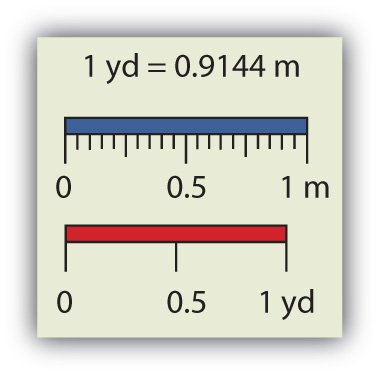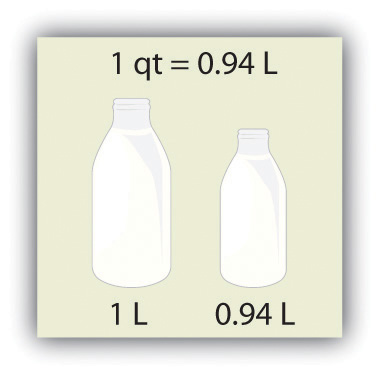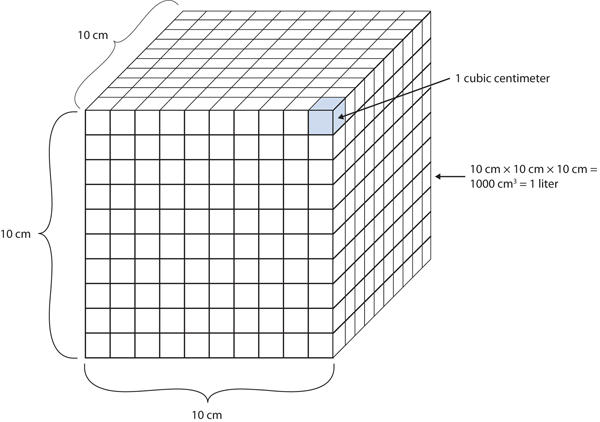Chapter 2. Measurements

# Expressing Units

### Learning Objectives

1. Learn the units that go with various quantities.
2. Express units using their abbreviations.
3. Make new units by combining numerical prefixes with units.

A number indicates “how much,” but the unit indicates “of what.” The “of what” is important when communicating a quantity. For example, if you were to ask a friend how close you are to Lake Erie and your friend says “six,” then your friend isn’t giving you complete information. Six what? Six miles? Six inches? Six city blocks? The actual distance to the lake depends on what units you use.

Chemistry, like most sciences, uses the International System of Units, or SI for short. (The letters SI stand for the French “le Système International d’unités.”) SI specifies certain units for various types of quantities, based on seven fundamental units for various quantities. We will use most of the fundamental units in chemistry. Initially, we will deal with three fundamental units. The meter (m) is the SI unit of length. It is a little longer than a yard (see Figure 2.3 “The Meter”). The SI unit of mass is the kilogram (kg), which is about 2.2 pounds (lb). The SI unit of time is the second (s).

Figure 2.3 The MeterThe SI standard unit of length, the meter, is a little longer than a yard.

To express a quantity, you need to combine a number with a unit. If you have a length that is 2.4 m, then you express that length as simply 2.4 m. A time of 15,000 s can be expressed as 1.5 × 104 s in scientific notation.

Sometimes, a given unit is not an appropriate size to easily express a quantity. For example, the width of a human hair is very small, and it doesn’t make much sense to express it in meters. SI also defines a series of numerical prefixes that refer to multiples or fractions of a fundamental unit to make a unit more conveniently sized for a specific quantity. Table 2.1 “Multiplicative Prefixes for SI Units” lists the prefixes, their abbreviations, and their multiplicative factors. Some of the prefixes, such as kilo-, mega-, and giga-, represent more than one of the fundamental unit, while other prefixes, such as centi-, milli-, and micro-, represent fractions of the original unit. Note, too, that once again we are using powers of 10. Each prefix is a multiple of or fraction of a power of 10.

Table 2.1 Multiplicative Prefixes for SI Units

Prefix Abbreviation Multiplicative Amount
giga- G 1,000,000,000 ×
mega- M 1,000,000 ×
kilo- k 1,000 ×
deci- d 1/10 ×
centi- c 1/100 ×
milli- m 1/1,000 ×
micro- μ* 1/1,000,000 ×
nano- n 1/1,000,000,000 ×
pico- p 1/1,000,000,000,000 ×
* The letter μ is the Greek letter lowercase equivalent to an m and is called “mu” (pronounced “myoo”).

To use the fractions to generate new units, simply combine the prefix with the unit itself; the abbreviation for the new unit is the combination of the abbreviation for the prefix and the abbreviation of the unit. For example, the kilometer (km) is 1,000 × meter, or 1,000 m. Thus, 5 kilometers (5 km) is equal to 5,000 m. Similarly, a millisecond (ms) is 1/1,000 × second, or one-thousandth of a second. Thus, 25 ms is 25 thousandths of a second. You will need to become proficient in combining prefixes and units. (You may recognize that one of our fundamental units, the kilogram, automatically has a prefix-unit combination, the kilogram. The word kilogram means 1,000 g.)

In addition to the fundamental units, SI also allows for derived units based on a fundamental unit or units. There are many derived units used in science. For example, the derived unit for area comes from the idea that area is defined as width times height. Because both width and height are lengths, they both have the fundamental unit of meter, so the unit of area is meter × meter, or meter2 (m2). This is sometimes spoken as “square meters.” A unit with a prefix can also be used to derive a unit for area, so we can also have cm2, mm2, or km2 as acceptable units for area.

Volume is defined as length times width times height, so it has units of meter × meter × meter or meter3 (m3), sometimes spoken as “cubic meters.” The cubic meter is a rather large unit, however, so another unit is defined that is somewhat more manageable: the liter (L). A liter is 1/1,000th of a cubic meter and is a little more than 1 quart in volume (see Figure 2.4 “The Liter”). Prefixes can also be used with the liter unit, so we can speak of milliliters (1/1,000th of a liter; mL) and kiloliters (1,000 L; kL).

Figure 2.4 The LiterThe SI unit of volume, the liter, is slightly larger than 1 quart.

Another definition of a liter is one-tenth of a meter cubed. Because one-tenth of a meter is 10 cm, then a liter is equal to 1,000 cm3 (Figure 2.5 “The Size of 1 Liter”). Because 1 L equals 1,000 mL, we conclude that 1 mL equals 1 cm3; thus, these units are interchangeable.

Figure 2.5 The Size of 1 LiterOne liter equals 1,000 cm3, so 1 cm3 is the same as 1 mL.

Units not only are multiplied together but also can be divided. For example, if you are traveling at one meter for every second of time elapsed, your velocity is 1 meter per second, or 1 m/s. The word per implies division, so velocity is determined by dividing a distance quantity by a time quantity. Other units for velocity include kilometers per hour (km/h) or even micrometers per nanosecond (μm/ns). Later, we will see other derived units that can be expressed as fractions.

### Example 2

1. A human hair has a diameter of about 6.0 × 10−5 m. Suggest an appropriate unit for this measurement and write the diameter of a human hair in terms of that unit.
2. What is the velocity of a car if it goes 25 m in 5.0 s?

Solution

1. The scientific notation 10−5 is close to 10−6, which defines the micro- prefix. Let us use micrometers as the unit for hair diameter. The number 6.0 × 10−5 can be written as 60 × 10−6, and a micrometer is 10−6 m, so the diameter of a human hair is about 60 μm.
2. If velocity is defined as a distance quantity divided by a time quantity, then velocity is 25 meters/5.0 seconds. Dividing the numbers gives us 25/5.0 = 5.0, and dividing the units gives us meters/second, or m/s. The velocity is 5.0 m/s.

Test Yourself

1. Express the volume of an Olympic-sized swimming pool, 2,500,000 L, in more appropriate units.
2. A common garden snail moves about 6.1 m in 30 min. What is its velocity in meters per minute (m/min)?

Answers

1. 2.5 ML
2. 0.203 m/min

### Key Takeaways

• Numbers tell “how much,” and units tell “of what.”
• Chemistry uses a set of fundamental units and derived units from SI units.
• Chemistry uses a set of prefixes that represent multiples or fractions of units.
• Units can be multiplied and divided to generate new units for quantities.

### Exercises

1. Identify the unit in each quantity.

a)  2 boxes of crayons

b)  3.5 grams of gold

2.  Identify the unit in each quantity.

a)  32 oz of cheddar cheese

b)  0.045 cm3 of water

3.  Identify the unit in each quantity.

a)  9.58 s (the current world record in the 100 m dash)

b)  6.14 m (the current world record in the pole vault)

4.  Identify the unit in each quantity.

a)  2 dozen eggs

b)  2.4 km/s (the escape velocity of the moon, which is the velocity you need at the surface to escape the moon’s gravity)

5.  Indicate what multiplier each prefix represents.

a)  k

b)  m

c)  M

6.  Indicate what multiplier each prefix represents.

a)  c

b)  G

c)  μ

7.  Give the prefix that represents each multiplier.

a)  1/1,000th ×

b)  1,000 ×

c)  1,000,000,000 ×

8.  Give the prefix that represents each multiplier.

a)  1/1,000,000,000th ×

b)  1/100th ×

c)  1,000,000 ×

9. Complete the following table with the missing information.

Unit Abbreviation
kilosecond
mL
Mg
centimeter

10.Complete the following table with the missing information.

Unit Abbreviation
kilometer per second
second
cm3
μL
nanosecond

11.  Express each quantity in a more appropriate unit. There may be more than one acceptable answer.

a)  3.44 × 10−6 s

b)  3,500 L

c)  0.045 m

12.  Express each quantity in a more appropriate unit. There may be more than one acceptable answer.

a)  0.000066 m/s (Hint: you need consider only the unit in the numerator.)

b)  4.66 × 106 s

c)  7,654 L

13.  Express each quantity in a more appropriate unit. There may be more than one acceptable answer.

a)  43,600 mL

b)  0.0000044 m

c)  1,438 ms

14.  Express each quantity in a more appropriate unit. There may be more than one acceptable answer.

a)  0.000000345 m3

b)  47,000,000 mm3

c)  0.00665 L

15.  Multiplicative prefixes are used for other units as well, such as computer memory. The basic unit of computer memory is the byte (b). What is the unit for one million bytes?

16.  You may have heard the terms microscale or nanoscale to represent the sizes of small objects. What units of length do you think are useful at these scales? What fractions of the fundamental unit of length are these units?

17.  Acceleration is defined as a change in velocity per time. Propose a unit for acceleration in terms of the fundamental SI units.

18.  Density is defined as the mass of an object divided by its volume. Propose a unit of density in terms of the fundamental SI units.

Answers

1.

a)  boxes of crayons

b)  grams of gold

3.

a)  seconds

b)  meters

5.

a)  1,000 ×

b)  1/1,000 ×

c)  1,000,000 ×

7.

a)  milli-

b)  kilo-

c)  giga-

9.

Unit Abbreviation
kilosecond ks
milliliter mL
megagram Mg
centimeter cm

11.

a)  3.44 μs

b)  3.5 kL

c)  4.5 cm

13.

a)  43.6 L

b)  4.4 µm

c)  1.438 s

15. megabytes (Mb)

17. meters/second2

## LicenseExpressing Units by Jessie A. Key is licensed under a Creative Commons Attribution-NonCommercial-ShareAlike 4.0 International License, except where otherwise noted.